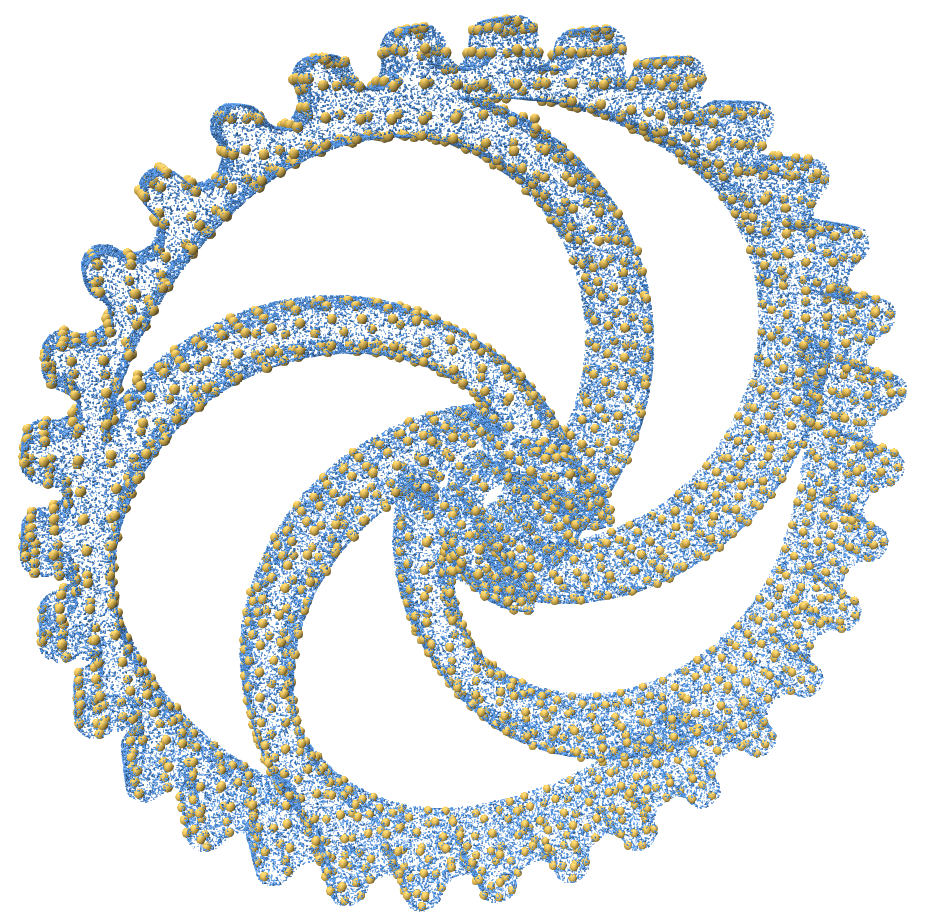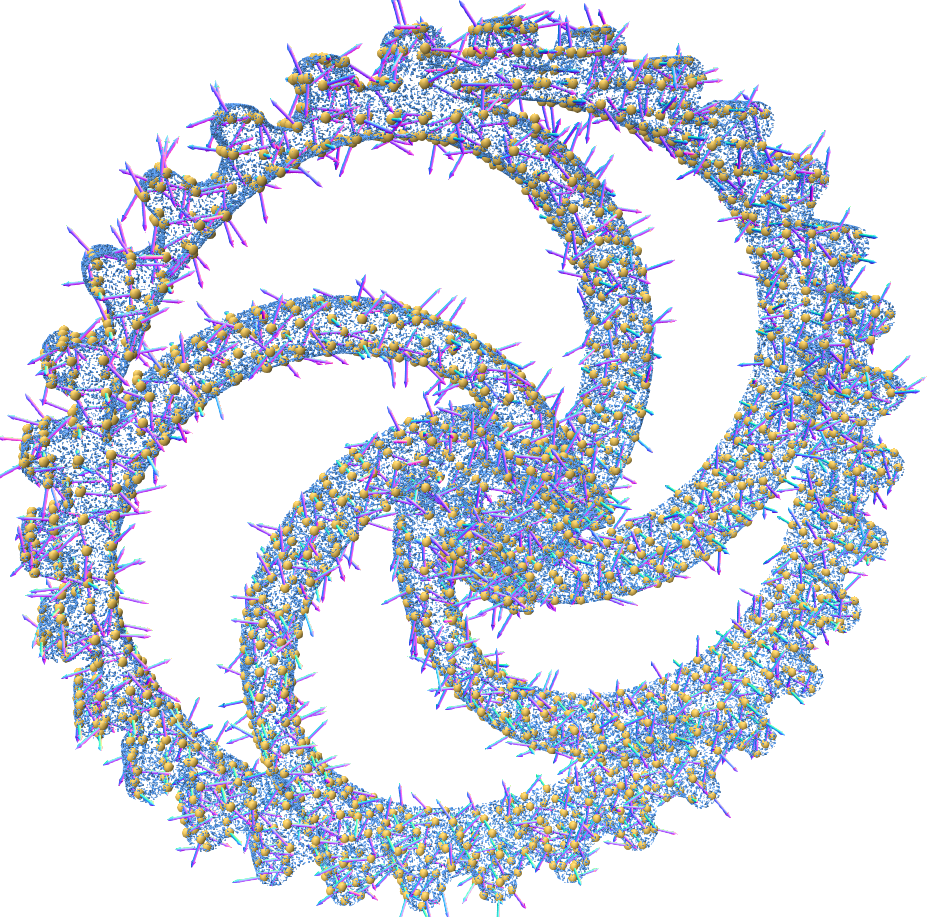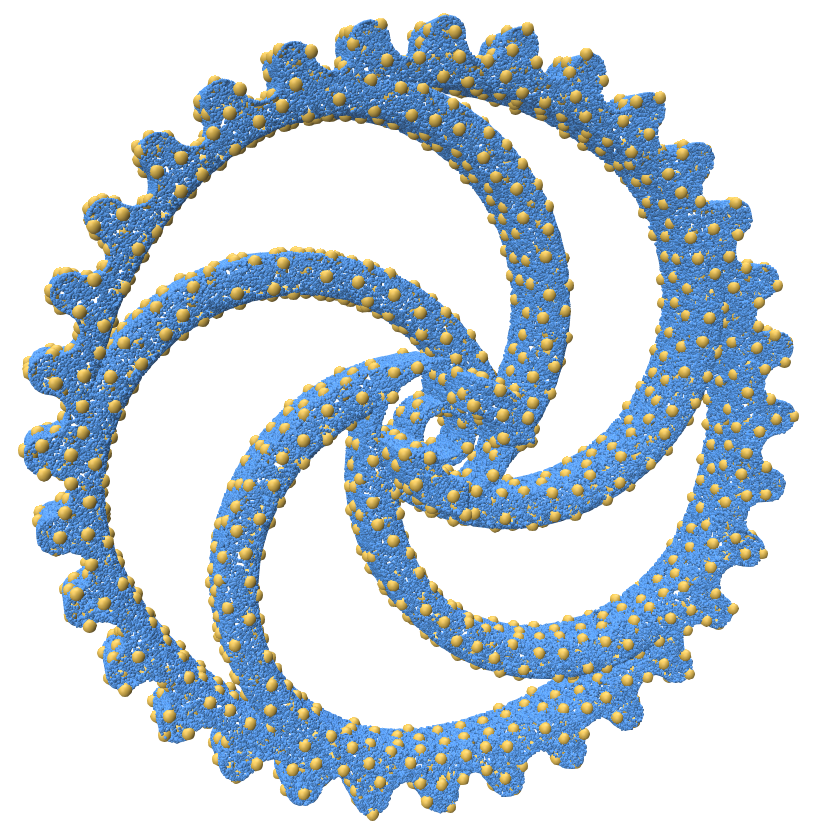# Resampling Point Clouds

Point Cloud Utils provides tools for re-sampling a point cloud in several ways.

Data in examples

In all the examples, below we first load the point cloud here

## Binning points in a voxel grid

In many 3D tasks, it is often advantageous to quantize a point cloud to a regular voxel grid in space. Such quantization can reduce the number of input points to an algorithm to match the resolution at which that algorithm operates.

Point Cloud Utils provides a simple function for quantizing a point cloud with arbitrary attributes into a voxel grid. Attributes are averaged within a voxel. The code below shows an example of this functionality with the figure showing the result.

import point_cloud_utils as pcu

# v is a [n, 3] shaped NumPy array of vertices
# n is a [n, 3] NumPy array of vertex normals

# We'll quantize our point cloud to a voxel grid with 128 voxels per axis
num_voxels_per_axis = 128

# Size of the axis aligned bounding box of the point cloud
bbox_size = p.max(0) - p.min(0)

# The size per-axis of a single voxel
sizeof_voxel = bbox_size / num_voxels_per_axis

# Downsample a point cloud on a voxel grid so there is at most one point per voxel.
# Any arguments after the points are treated as attribute arrays and get averaged within each voxel
v_sampled, n_sampled = pcu.downsample_point_cloud_on_voxel_grid(sizeof_voxel, p, n)## Downsampling a point cloud to have a blue noise distribution

If we have a dense point cloud, we may want to downsample it to sparse point cloud where all points are about evenly spaced apart. Such a distribution of points is called a "blue noise" distribution. Formally, this means that the expected distance between points on the surface is some constant. i.e. for a point cloud $$P$$: $$\mathbb{E}_{x \in P} ||x - \text{nearest_neighbor}(x)|| = \epsilon$$

This can be achieved with Poisson Disk Sampling. Point cloud utils supports downsampling a point cloud to a target radius or to a target number of points

Downsampling a points to a blue noise distribution with a target number of points

import point_cloud_utils as pcu
import numpy as np

# v is a [n, 3] shaped NumPy array of vertices
# n is a [n, 3] NumPy array of vertex normals

### Option 1:
### Downsampling a points to a blue noise distribution with a target number of points
# idx is an array of integer indices into v indicating which samples to keep
target_num_pts= int(0.1*p.shape)  # 10% of the number of input points
idx = pcu.downsample_point_cloud_poisson_disk(p, num_samples=target_num_pts)

### Option 2:
### Downsampling a points to a blue noise distribution with a target radius
# idx is an array of integer indices into v indicating which samples to keep
target_radius = np.linalg.norm(p.max(0) - p.min(0)) * 0.02  # 2% of the bounding box radius

# Use the indices to get the sample positions and normals
v_sampled = p[idx]
n_sampled = n[idx]## Deduplicating a point cloud

You can deduplicate a point cloud by removing vertices that are equal up to some threshold. The example below removes duplicate points with a threshold of $$10^{-1}$$

import point_cloud_utils as pcu

# p is a (n, 3)-shaped array of points (one per row)
# p is a (n, 3)-shaped array of normals at each point